# Civil Engineering - Strength of Materials

### Exercise :: Strength of Materials - Section 3

1.

The ratio of the moments of resistance of a solid circular shaft of diameter D and a hollow shaft (external diameter D and internal diameter d), is

 A.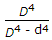B.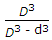C.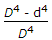D.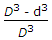E. none of these.

Explanation:

No answer description available for this question. Let us discuss.

2.

A cantilever carries is uniformly distributed load W over its whole length and a force W acts at its free end upward. The net deflection of the free end will be

 A. zero B.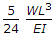upward C.downward D. none of these.

Explanation:

No answer description available for this question. Let us discuss.

3.

The moment diagram for a cantilever carrying a concentrated load at its free end, will be

 A. triangle B. rectangle C. parabola D. cubic parabola.

Explanation:

No answer description available for this question. Let us discuss.

4.

In a shaft rotated by a couple, the shear force varies

 A. from zero at the centre to a maximum at the circumference B. from minimum at the centre of maximum at the circumference C. from maximum at the centre to zero at the circumference D. equally throughout the section E. none of these.

Explanation:

No answer description available for this question. Let us discuss.

5.

The section modulus of a rectangular light beam 25 metres long is 12.500 cm3. The beam is simply supported at its ends and carries a longitudinal axial tensile load of 10 tonnes in addition to a point load of 4 tonnes at the centre. The maximum stress in the bottom most fibre at the mid span section, is

 A. 13.33 kg/cm2 tensile B. 13.33 kg/cm2 compressive C. 26.67 kg/cm2 tensile D. 26.67 kg/cm2 compressive E. none of these.